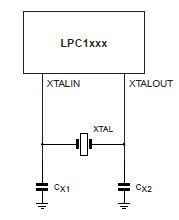## How to calculate the value of crystal load capacitors?

cancel
Showing results for
Did you mean:

## How to calculate the value of crystal load capacitors?

91,541 Views
Senior Contributor I

The following formula may be used to calculate a parallel resonant crystal's external load capacitors:
CL = ((CX1 x CX2) / (CX1 + CX2)) + Cstray
where:
CL = the crystal load capacitance
Cstray = the stray capacitance in the oscillator circuit, which will normally be in the 2pF to 5pF range.

Assuming that CX1=CX2 then the equation becomes:
CL = ((CX1 x CX1) / (2 x CX1)) + Cstray
CL = (CX1 / 2) + Cstray
Rearranging the equation, we can find the external load capacitor value:
CX1 = 2(CL - Cstray)

For example, if the crystal load capacitance is 15pF, and assuming Cstray=2pF, then:
CX1 = CX2 = 2(15pF - 2pF) = 26pF

It is difficult to know exactly what the stray capacitance is, but if you find the oscillation frequency is too high, the load capacitor values can be increased. If the frequency is too low, the load capacitors can be decreased.
The device data sheet may also define a maximum crystal series resistance Rs.Labels (20)

• ### lpc800

2 Replies
2,473 Views
Senior Contributor I

#2Submitted by larsen on Thu, 2014-11-06 03:39.

atmel defines the stray capacity as being from xtal terminal to gnd.
NXP defines the stray capacity as parallel to the crystal. This gives

Stray capacities: Catmel =2 Cnxp

so it is all a matter of definition. Anyway there are typically some "handwaving" involved in stray estimates anyway.

2,473 Views
Senior Contributor I
 #1Submitted by ericll75 on Fri, 2014-03-28 12:38.According Atmel AVR042, p.12, the equation for the external capacitors is C = 2CL - Cstray. The same thing is reflected in Atmel AT91 ARM Thumb app note (1740A), p.7.Why the difference in equations? Why does Atmel put the stray capacitance value into CX1 or CX2 (according to AVR042, CX1 = C + Cstray), whereas the equation here puts Cstray as a separate term?Am I missing something?